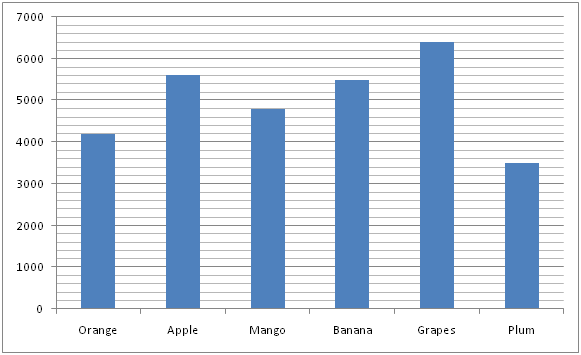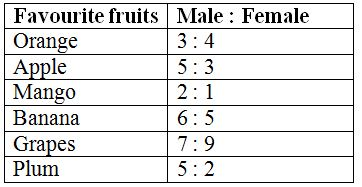# gkrecall

We brings you daily Current affairs, daily Current Affairs Quiz, weekly Current Affairs, weekly Current Affairs Quiz to enhance your preparation for upcoming exams. We also provide articles related to quant, reasoning, English, ssc etc .

## QUANT PRACTICE SET 6 FOR RRB PO & CLERK PRELIM 2020

RRB Clerk and RRB PO Prelims exam are going to be held in the upcoming months. We have already provided you with the PDFs of many topics of Quantitative Aptitude like Simplification/Approximation, Number Series,datainterpretation.important Arithmetic questions to prepare for RRB Clerk and PO Pre exam. Practicing these questions will help you to know about the level of the questions. To increase your speed and accuracy, enhance your calculations.

### START QUIZ

1) A man can row a certain distance downstream in 12 hours and come back in 20 hours, then what is the speed of the boat if the speed of the stream is 3 km/hr?
a) 20 km/hr
b) 18 km/hr
c) 12 km/hr
d) 17 km/hr
e) 13 km/hr
2) Megala, Sruthi and Nandhini enter into a business. Megala invested some amount in the starting. After 1 year, Sruthi invested double the amount invested by Megala and after 1 year 6 months, Nandhini invested thrice the amount invested Megala. They earn a profit of Rs 330000 at the end of 2 years. What is the share of Nandhini?
a) Rs 15000
b) Rs 75000
c) Rs 85000
d) Rs 46000
e) Rs 90000
3) The cost of five chairs and three tables is Rs 4440. Cost of one chair is Rs 320 less than cost of one table. What is the cost of two tables and two chairs?
a) Rs 3760
b) Rs 4670
c) Rs 6890
d) Rs 2380
e) Rs 7070
4) A train running at the speed of 48m/s crosses a pole in 40 seconds less than the time it requires to cross a platform thrice its own length at the same speed. What is the length of the train?
a) 640 seconds
b) 479 seconds
c) 356 seconds
d) 245 seconds
e) 648 seconds
5) A bag contains an equal number of 50 paisa, 25 paisa, 20 paisa and 5 paisa coins respectively. If the total amount is Rs 50, how many coins of each type are there in the bag?
a) 67
b) 35
c) 48
d) 24
e) 50
Directions (Q. 6 – 10): Study the following information carefully and answer the given questions?
The following bar graph shows the total number of peoples having 6 differentfavourite fruits in certain village.The table shows the ratio of male to that of female having those favourite fruits in the village.6) Find the ratio between the total number of male having a favourite fruit of Orange to that of total number of female having a favourite fruit of Plum?
a) 11 : 7
b) 9 : 5
c) 23 : 19
d) 37 : 28
e) None of these
7) Find the difference between the total number of male having a favourite fruits of Apple and Grapes together to that of total number of female having a favourite fruits of Mango and Banana together?
a) 2800
b) 3400
c) 3600
d) 2200
e) None of these
8) Find the average number of female having a favourite fruits of Apple, Mango, Banana and Grapes together?
a) 2450
b) 2600
c) 2250
d) 2700
e) None of these
9) Total number of peoples having a favourite fruits of Orange and Plum together is approximately what percentage of total number of peoples having a favourite fruits of Mango and Banana together?
a) 105 %
b) 120 %
c) 75 %
d) 60 %
e) 90 %
10) Total number of females having a favourite fruit of Plum is approximately what percentage less than the total number of males having a favourite fruit of Apple?
a) 86 %
b) 71 %
c) 57 %
d) 103 %
e) 120 %
SOLUTIONS
Direction (1-5) :
Let the total distance travelled by D kms and the speed of the boat be S km/hr.
Then upstream speed= (S-3) km/hr
And downstream speed= (S+3) km/hr
(S-3)*20 = (S+3)* 12
20S-60 = 12S+36
8S = 96
S=12 km/hr
Thus speed of the boat= 12 km/hr
Let the amount invested by Megala be Rs x.
Then Sruthi’s investment be 2x and Nandhini’s investment be 3x.
Thus ratio of investment by all of them in 2 years= x*24:2x*12:3x*6
= 24x: 24x: 18x
=4:4:3
Thus share of Nandhini= 3/11*330000
=Rs 90000
If the cost price of 1 chair Rs x then CP of 1 table=Rs (x+320)
Thus 5x+3(x+320) = 4440
5x+3x+960= 4440
8x = 3480
x=435
Then CP of two tables and two chairs= 2(x+x+320)
= 4x+640
= 4*435+640
= Rs 2380
Let the length of the train be x m.
Then length of the platform=3x m
Now time taken by the train to cross platform=(x+3x)/48
= 4x/48 seconds
And time taken by the train to cross the pole= x/48 seconds
Then, (4x/48)-(x/48)=40
3x/48=40
x=640 seconds
Let the number of coins in the bag be x
Then, 0.5x+0.25x+0.2x+0.05x= 50
x= 50
Direction (6-10) :
The total number of male having a favourite fruit of Orange
= >4200*(3/7)
The total number of female having a favourite fruit of Plum
= >3500*(2/7)
Required ratio = [4200*(3/7)] : [3500*(2/7)] = 9 : 5
The total number of male having a favourite fruits of Apple and Grapes together
= >5600*(5/8) + 6400*(7/16)
= > 3500 + 2800 = 6300
The total number of female having a favourite fruits of Mango and Banana together
= >4800*(1/3) + 5500*(5/11)
= > 1600 + 2500 = 4100
Required difference = 6300 – 4100 = 2200
The average number of female having a favourite fruits of Apple, Mango, Banana and Grapes together
= >[5600*(3/8) + 4800*(1/3) + 5500*(5/11) + 6400*(9/16)] / 4
= > [2100 + 1600 + 2500 + 3600] / 4
= > 9800 / 4 = 2450